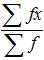# When does mean have meaning? via @CambridgeMaths

The humble arithmetic ‘mean’, a calculation that primary school students are expected to be able to access, is a microcosm of the key ideas of statistics with links to wider mathematics. It can be taught as a mathematical process in which students “add up all the values and divide by how many there are” and as a formulaBut if students’ only experience of the mean is to calculate a value from a set of numbers, they miss out on the multitude of lines of mathematical enquiry captured in this deceptively simple statistic.

So when is a mean actually meaningful? Everyone has pondered over the idea of the average UK family comprising 2.4 children and wondered how one should process the idea of .4 of a child (although this figure has decreased since the 90s sitcom that brought the value into the public consciousness – the latest stats show it is around 1.7) . This relatively common issue of applying the mean to discrete data is often encountered and can be interpreted as suggesting that a family “usually” has either two or three children.

There are numerous other instances in which it is technically possible to calculate the arithmetic mean of a set of numbers, but the value of the result provides no useable information. Using a sporting example, what does the mean of the player numbers imply for Portsmouth football club? Each player wears a number, but those numbers do not represent any mathematical information so the mean that is produced tells us nothing about the team; adding them up isn’t at all useful.

This does not always have to be the case though, if each player in the first team was numbered from 1 to 11, with any subs numbered differently, we could infer if the team was playing its “strongest side”. The mean of the consecutive numbers 1 to 11 is 6, so if we found the mean of the shirts for the players on the pitch to be larger than 6 we could infer that the team was not playing its best XI. While this does now tell us something about the team, we could infer the same without calculation simply by looking at the players on the pitch and noticing that one of the shirt numbers was greater than 11. As mathematicians, we should all be fans of the most efficient method!

Another example would be to calculate the mean weight of lions in a zoo. Again technically possible given a sufficiently fearless zookeeper and a large enough scale, but to what end? As a measure, it has no comparative value. The precise balance of old animals to young, and male to female, is likely to be zoo specific. In contrast, calculating the mean weight of adult male lions and the mean weight of adult female lions allows a sensible comparative value when a new animal arrives at the zoo. The new lion can meaningfully be compared to the mean weight of similar lions allowing keepers to infer whether the animal is a typical example of its species, in contrast comparing it to the mean weight of lions currently in the zoo yields nothing obviously useful.

How about the mean of the coordinates that define the vertices of a 2D shape? Under certain circumstances, this will give the centre of mass of the object. Here the mean is no longer technically a statistical calculation as there is no underlying variation in the figure being measured, but it does provide important additional information about the object’s structure.

Clearly, when deciding if the mean is appropriate, consideration needs to be given to the underlying data. Is it discrete or continuous? Is the underlying measurement scale nominal, ordinal or interval? Is like being compared with like?

A deep understanding of the mean as a meaningful calculation can only be achieved if learners have repeated opportunities to consider in detail the context of the data before attempting to interpret what the mean value represents under different constraints. Why not try exploring ideas around the meaning of the mean with your classes and let us know how you get on!